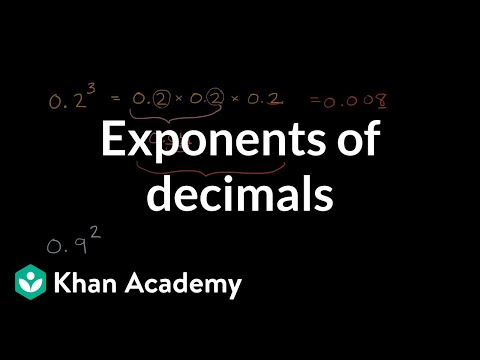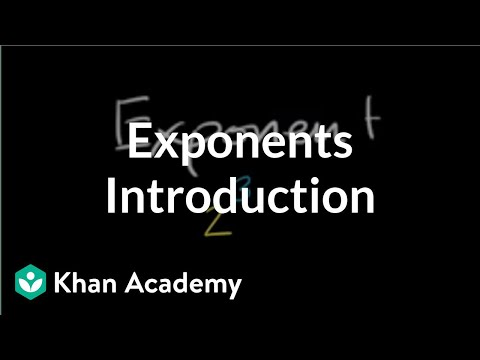Video

# The zeroth power (Full video)

Description: Learn why a number raised to the zero power equals 1. If we think about something like two to the third power, we could view this as taking three twos and multiplying them together, so two times two times two, or equivalently, we could say this is the same thing as taking a one and then multiplying it by two three times, so actually, let's just go with this definition right over here, and this, of course, is going to be equal to eight. Based on this definition of what an exponent is, what would two to the zeroth power be? Well, the way we just talked about it, we just said exponentiation is you start with a one and you multiply it by the base zero times, so we're not gonna multiply it by any two, so we're just gonna be left with a one.

### Other videos you might be interested in### Exponents of decimals (Full video)